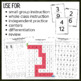# Equivalent Fractions Centers and Activities: A Fun Unit to Build UnderstandingSubject
Resource Type
Format
PDF (6 MB|54 pages)
Standards
\$6.75

#### Also included in

1. This mega fractions bundle contains 7 of my fraction resources, covering everything from understanding 1/2, to fractions on a number line, to equivalent fractions, to comparing fractions. Save over 30% when you buy the mega bundle!This resource contains: Understanding the Fraction 1/2This resource
\$18.50
\$27.25
Save \$8.75

### Description

Help make teaching equivalent fractions easier and more understandable for your students - This unit starts with a basic introduction to equivalent fractions, and then is scaffolded with visuals to help your students master this skill as the concepts get more difficult. The scaffolding also helps you differentiate more easily. Activities, centers, and games are provided for additional equivalent fractions practice. And as an added bonus, most of these activities are no prep equivalent fraction resources - just print and go!

Save over 30% when you buy this as part of my Fractions: Mega Bundle which includes resources to practice fractions on a number line, comparing fractions, and more.

This resource includes:

-10 equivalent fraction worksheets that help students develop a true understanding of equivalent fractions

-a "Find the Equivalent Fraction" Scavenger Hunt activity

-4 fun mazes that can be used as no prep centers

-a "snowball fight" whole class activity

-3 equivalent fraction coloring mystery pictures that make great centers

These activities can be used in upper elementary for small groups, assessments/test, center activities, explicit instruction, math workshop, for special education students, for equivalent fraction stations / workstations, and more.

This directly addresses common core standards: 3.NF.A.3.A, 3.NF.A.3.B, and 4.NF.A.1 for 3rd grade and 4th grade students. They make a great equivalent fraction review for 5th grade students.

Check out the preview everything included in this resource!

You might also be interested in:

Fractions on a Number Line

Multi Step Word Problems

Comparing Fractions to 1/2

Total Pages
54 pages
Included
Teaching Duration
N/A
Report this Resource to TpT
Reported resources will be reviewed by our team. Report this resource to let us know if this resource violates TpT’s content guidelines.

### Standards

to see state-specific standards (only available in the US).
Compare two fractions with different numerators and different denominators, e.g., by creating common denominators or numerators, or by comparing to a benchmark fraction such as 1/2. Recognize that comparisons are valid only when the two fractions refer to the same whole. Record the results of comparisons with symbols >, =, or <, and justify the conclusions, e.g., by using a visual fraction model.
Explain why a fraction 𝘢/𝘣 is equivalent to a fraction (𝘯 × 𝘢)/(𝘯 × 𝘣) by using visual fraction models, with attention to how the number and size of the parts differ even though the two fractions themselves are the same size. Use this principle to recognize and generate equivalent fractions.
Recognize and generate simple equivalent fractions, (e.g., 1/2 = 2/4, 4/6 = 2/3). Explain why the fractions are equivalent, e.g., by using a visual fraction model.
Understand two fractions as equivalent (equal) if they are the same size, or the same point on a number line.

### Q & A

Teachers Pay Teachers is an online marketplace where teachers buy and sell original educational materials.